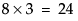1 Select Help > Sample Data Library and open Cheese.jmp.
 2 Select Analyze > Fit Model.
 3 Select Response and click Y.
 4 Select Cheese and click Add.
 5 Select Count and click Freq.
 6 Click Run.
Ordinal Logistic Fit Report
The model fit in this example reduces the –LogLikelihood of 429.9 for the intercept-only model to 355.67 for the full model. This reduction yields a likelihood ratio chi-square statistic for the whole model of 148.45 with 3 degrees of freedom. Therefore, the difference in perceived cheese taste is highly significant.
 Cheese Estimate Preference A –0.8622 2nd place B 2.4896 least liked C 0.8477 3rd place D –2.4750 most liked
As an ordinal problem, each of the first eight response levels has an intercept, but there are only three parameters for the four levels of Cheese. As a result, there are 3 degrees of freedom in the ordinal model. The ordinal model is the Fitted model in the Lack of Fit test.
There are 28 rows with a nonzero value of Count in the data table, so there are 28 - 4 = 24 replicated points with respect to the levels of Cheese. Therefore, the Saturated model in the Lack of Fit test has 24 degrees of freedom.
As a nominal problem, each of the first eight response levels has an intercept as well as three parameters for the four levels of Cheese. As a result, there aredegrees of freedom in the nominal model. Therefore, the nominal model is the Saturated model in the Lack of Fit test.

Help created on 9/19/2017